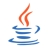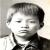# 哈 ，喷下 static 我的个人观点

@乌龟壳 我专门喷个水帖，讨论下static吧。算个人片面观点，抛砖引玉，欢迎大家指正。

static ，基本的三个用法，针对数据（我的个人习惯用语，可等同为C语言教科书上的变量），有 函数内的，和函数外的。 另一个用法是针对函数本身的。

```static void func(void){
return ;
} ```

```static signed char inc(signed char a){
return a+1;
}

signed char g_inc(signed char a){
if ((a+1) < a){
return a;
}else{
return a+1;
}
}```

000#### 引用来自“乌龟壳”的评论

0#### 引用来自“AutoPlus”的评论

0```package org.wuguike.utils

class P {
public int x;
private int y;
public static int z = 1;
}

package org.wuguike.helper

class P {
public int x;
private int y;
public static int z = 2;
}

package org.wuguike.test

class P {
public static void Main(){
printf(org.wuguike.utils.P.z);
printf(org.wuguike.helper.P.z);
}
}```0#### 引用来自“乌龟壳”的评论

```package org.wuguike.utils

class P {
public int x;
private int y;
public static int z = 1;
}

package org.wuguike.helper

class P {
public int x;
private int y;
public static int z = 2;
}

package org.wuguike.test

class P {
public static void Main(){
printf(org.wuguike.utils.P.z);
printf(org.wuguike.helper.P.z);
}
}```

C 的 static 目的是防止外部文件引用本文件，造成命名冲突；另外，就是提供一个共享实例，全进程/线程只有一个对象。最常用的就是静态变量：

```static struct P *p = null;
struct P *getP() {
if (p == null) p = malloc(...);
return p;
}```

Java 的 private public 才是相当于 C 的 static extern 目的。如果要制造 C 的 static 静态变量目的（C 并不关心 struct 的字段可不可以隐藏，C 的哲学是程序员应该具备关心成员的本分），应该相当于：

```public class P {
private P() {}
private static P instance = null;
public static P getInstance() {
if (instance == null) {
instance = new P();
}
return instance;
}
}

{
P p1 = P.getInstance();
P p2 = P.getInstance();
}```